Скачать презентацию Electrostatic Field • Electric charges, interaction of

electrostatics-new.ppt

• Размер: 339 Кб
• Количество слайдов: 20

## Описание презентации Electrostatic Field • Electric charges, interaction of по слайдам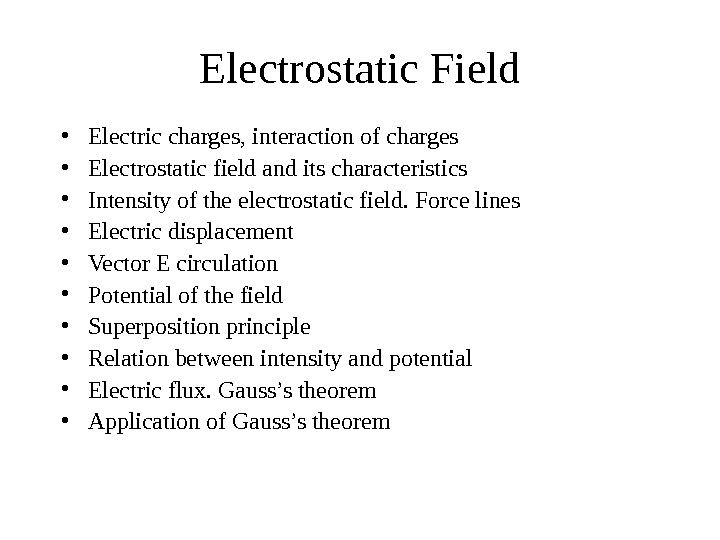Electrostatic Field • Electric charges, interaction of charges • Electrostatic field and its characteristics • Intensity of the electrostatic field. Force lines • Electric displacement • Vector E circulation • Potential of the field • Superposition principle • Relation between intensity and potential • Electric flux. Gauss’s theorem • Application of Gauss’s theorem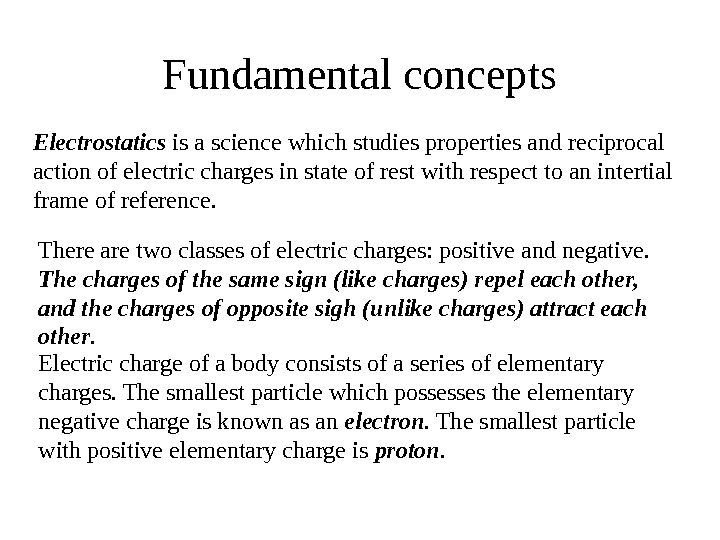Fundamental concepts Electrostatics is a science which studies properties and reciprocal action of electric charges in state of rest with respect to an intertial frame of reference. Electric charge of a body consists of a series of elementary charges. The smallest particle which possesses the elementary negative charge is known as an electron. The smallest particle with positive elementary charge is proton. There are two classes of electric charges: positive and negative. The charges of the same sign (like charges) repel each other, and the charges of opposite sigh (unlike charges) attract each other.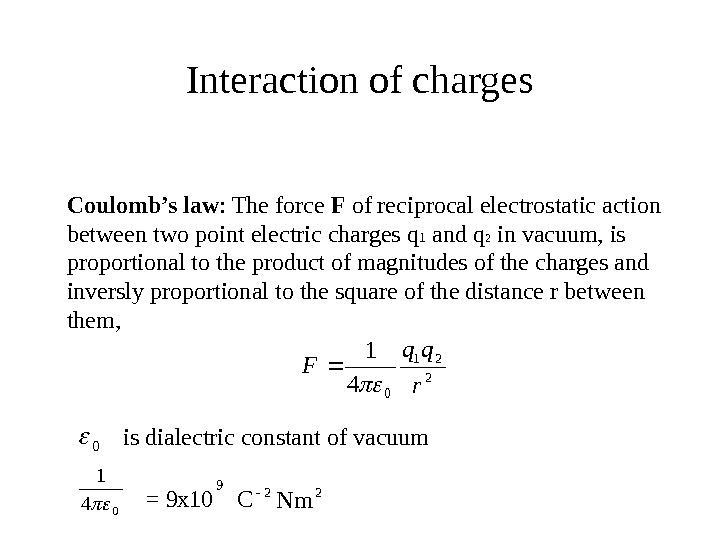Interaction of charges 2 21 04 1 r qq F Coulomb’s law : The force F of reciprocal electrostatic action between two point electric charges q 1 and q 2 in vacuum, is proportional to the product of magnitudes of the charges and inversly proportional to the square of the distance r between them, 0 is dialectric constant of vacuum 04 1 = 9 x 10 C 922 Nm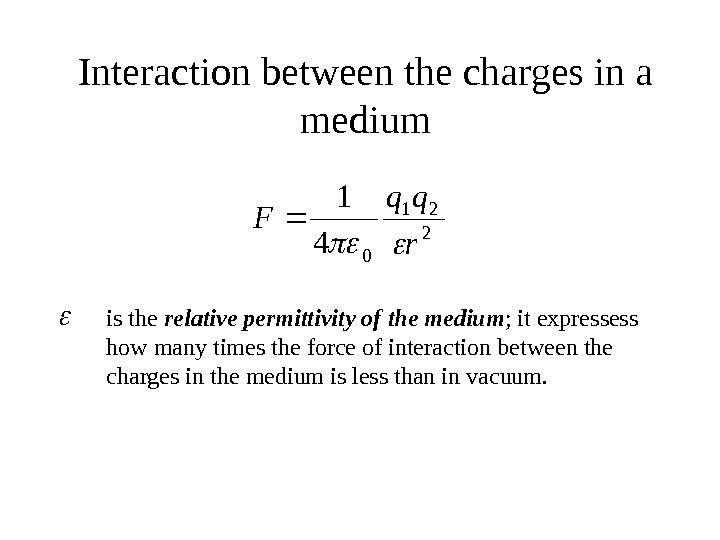Interaction between the charges in a medium 2 21 04 1 r qq F is the relative permittivity of the medium ; it expressess how many times the force of interaction between the charges in the medium is less than in vacuum.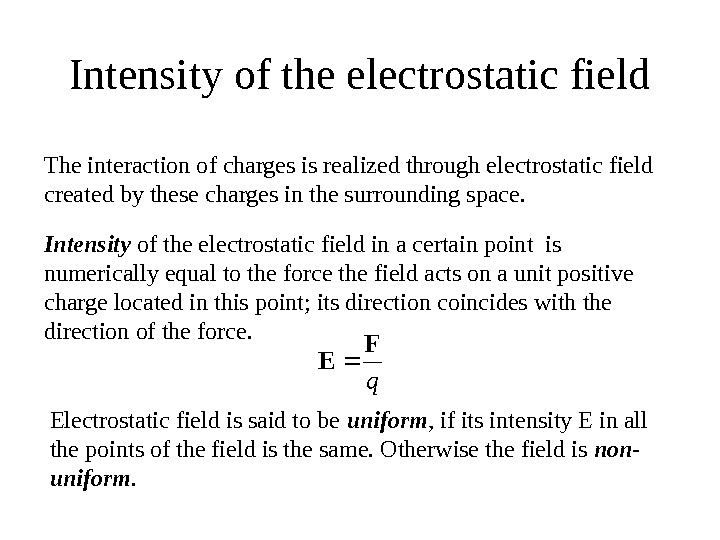Intensity of the electrostatic field q. F E Intensity of the electrostatic field in a certain point is numerically equal to the force the field acts on a unit positive charge located in this point; its direction coincides with the direction of the force. Electrostatic field is said to be uniform , if its intensity E in all the points of the field is the same. Otherwise the field is non- uniform. The interaction of charges is realized through electrostatic field created by these charges in the surrounding space.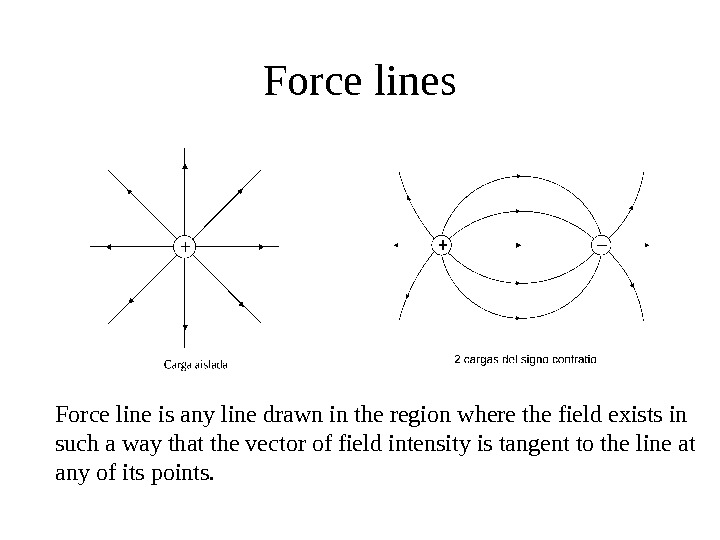Force lines Force line is any line drawn in the region where the field exists in such a way that the vector of field intensity is tangent to the line at any of its points.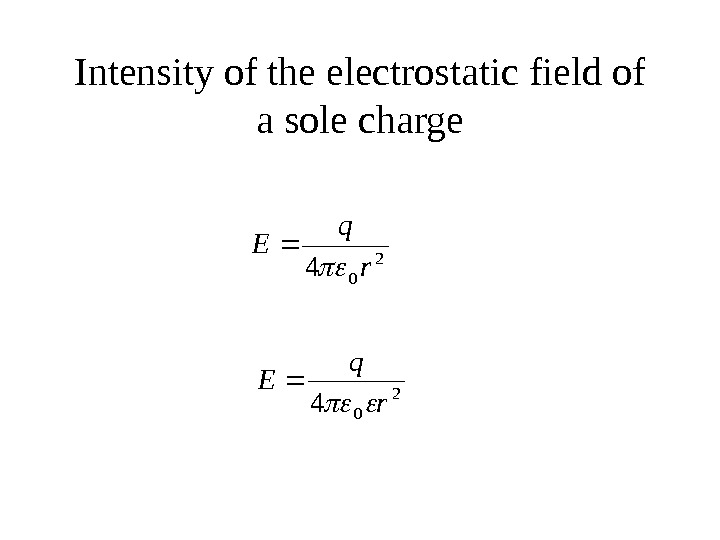Intensity of the electrostatic field of a sole charge 2 04 r q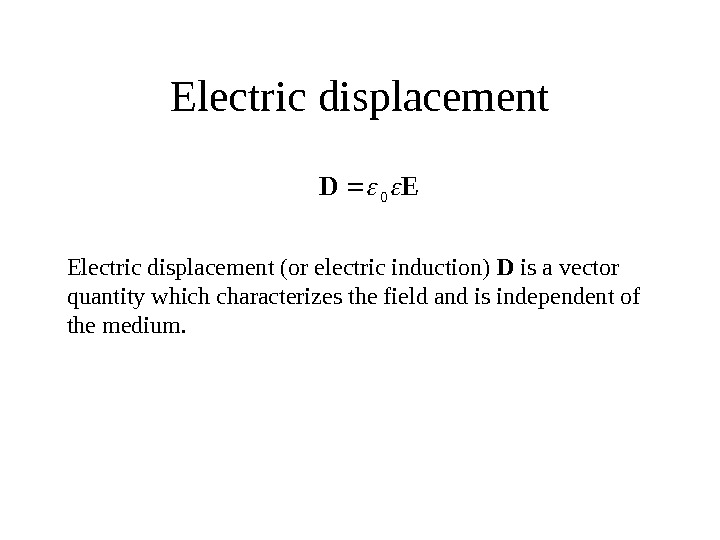Electric displacement. ED 0 Electric displacement (or electric induction) D is a vector quantity which characterizes the field and is independent of the medium.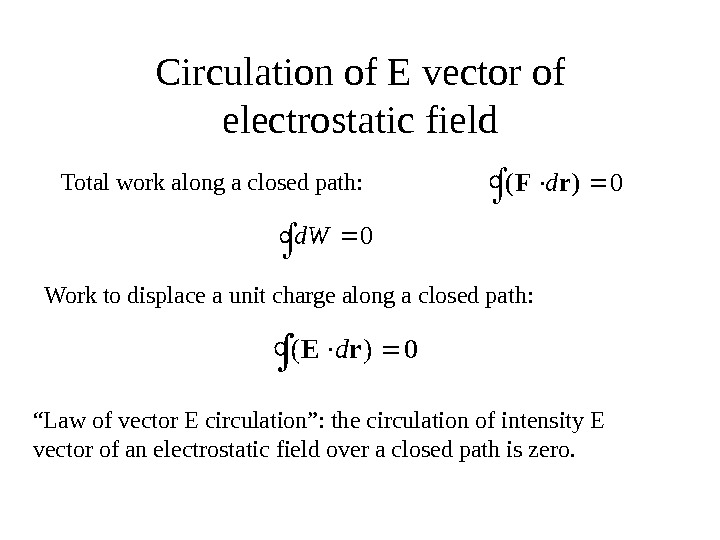Circulation of E vector of electrostatic field 0 d. W ()Frd 0 Total work along a closed path: Work to displace a unit charge along a closed path: ()Erd 0 “ Law of vector E circulation”: the circulation of intensity E vector of an electrostatic field over a closed path is zero.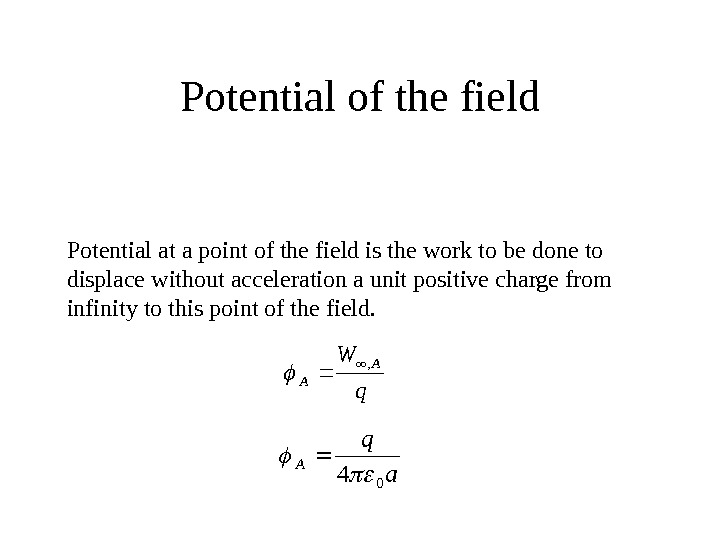Potential of the field Potential at a point of the field is the work to be done to displace without acceleration a unit positive charge from infinity to this point of the field. q WA A , a q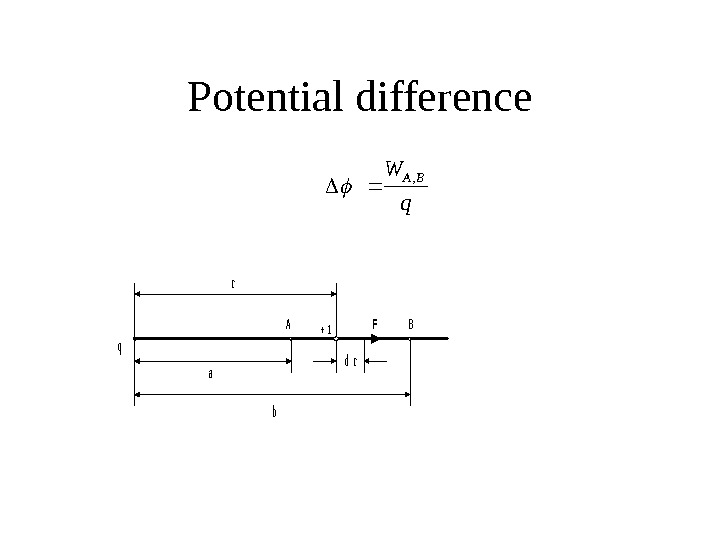Potential difference. ABF a b r + 1 q dr q WВА,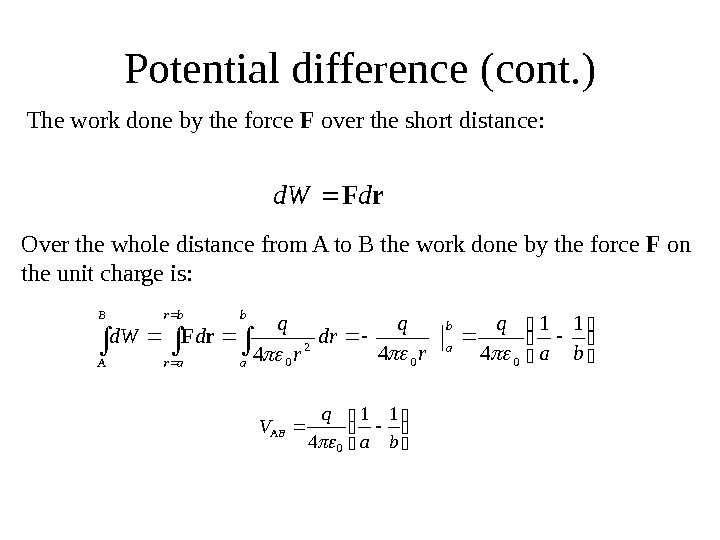Potential difference (cont. )r. Fdd. W The work done by the force F over the short distance: ba q r q dr r q dd. W b a br ar B A 11 44400 2 0 r. F Over the whole distance from A to B the work done by the force F on the unit charge is: ba q V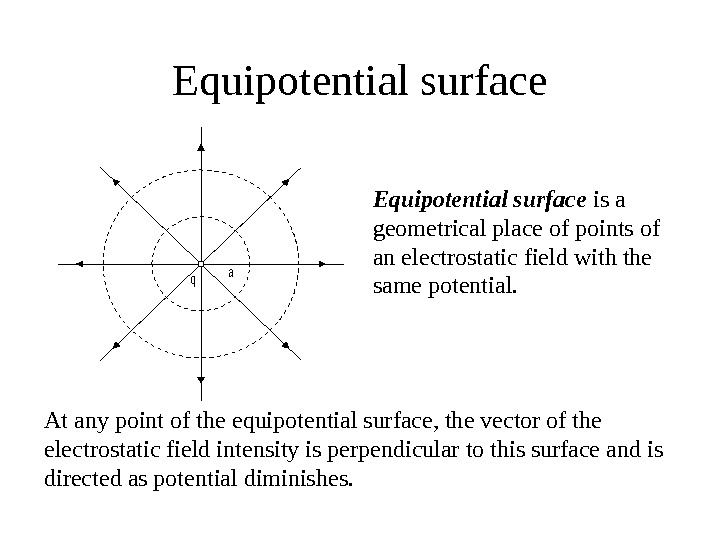Equipotential surfaceqa Equipotential surface is a geometrical place of points of an electrostatic field with the same potential. At any point of the equipotential surface, the vector of the electrostatic field intensity is perpendicular to this surface and is directed as potential diminishes.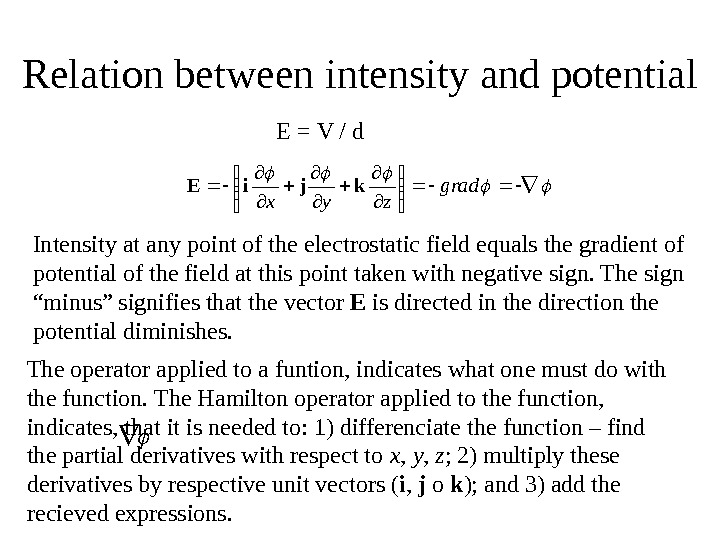Relation between intensity and potential grad zyx kji. E The operator applied to a funtion, indicates what one must do with the function. The Hamilton operator applied to the function, indicates, that it is needed to: 1) differenciate the function – find the partial derivatives with respect to x , y , z ; 2) multiply these derivatives by respective unit vectors ( i , j o k ); and 3) add the recieved expressions. E = V / d Intensity at any point of the electrostatic field equals the gradient of potential of the field at this point taken with negative sign. The sign “minus” signifies that the vector E is directed in the direction the potential diminishes.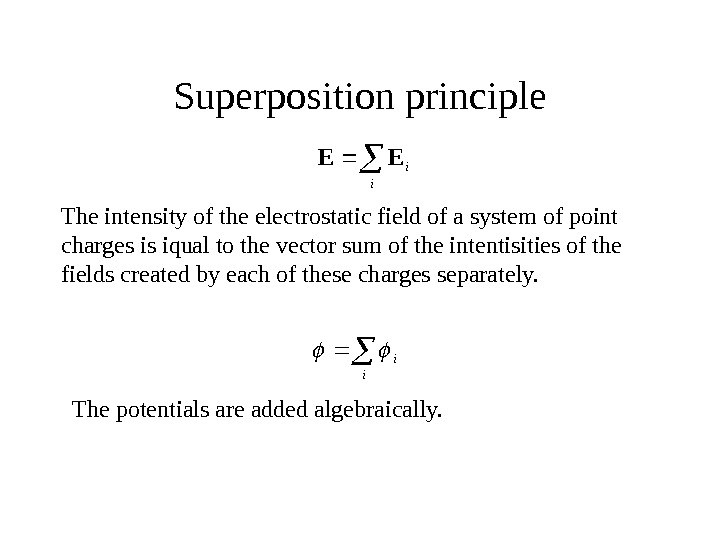Superposition principle The intensity of the electrostatic field of a system of point charges is iqual to the vector sum of the intentisities of the fields created by each of these charges separately. i i. EE i i The potentials are added algebraically.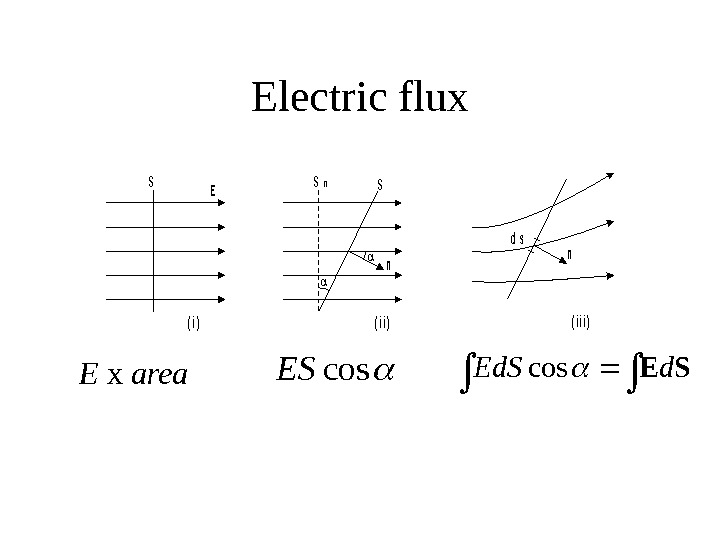Electric flux. SE ( i )( i i ) n Sn. S n d s ( i i i ) cos. ES E x area SEd. Scos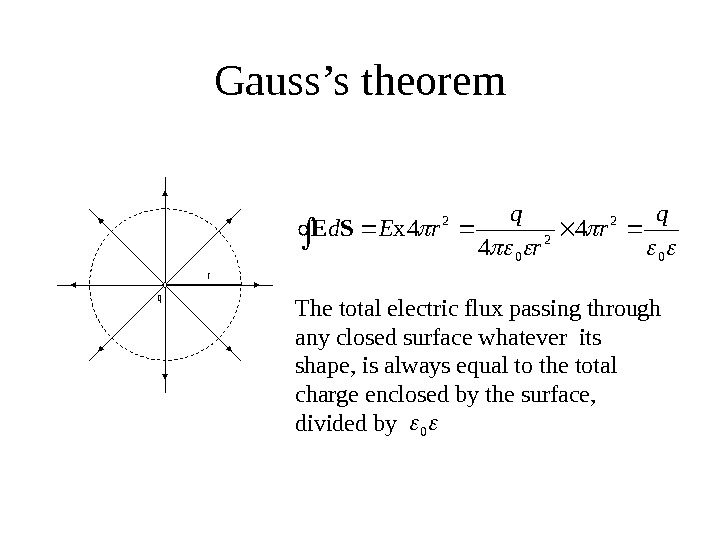Gauss’s theoremq r 0 2 2 0 2 4 4 4 x q r r q r. Ed. SE The total electric flux passing through any closed surface whatever its shape, is always equal to the total charge enclosed by the surface, divided by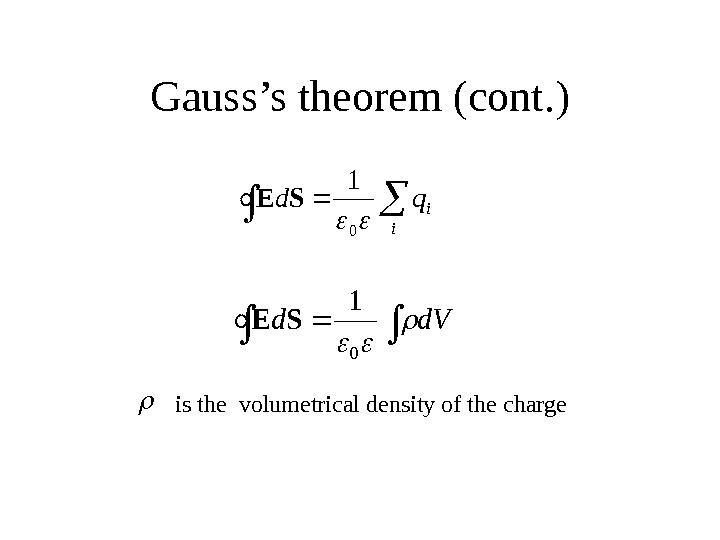Gauss’s theorem (cont. ) i iqd 0 1 SE d. Vd 0 1 SE is the volumetrical density of the charge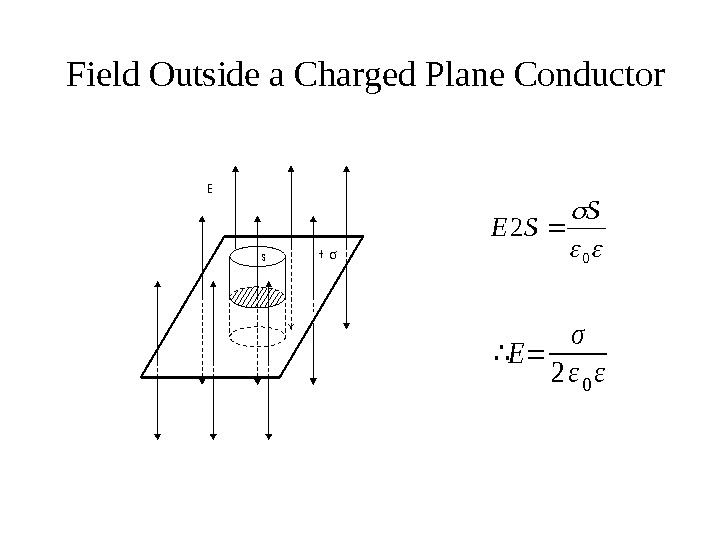Field Outside a Charged Plane Conductors+ E 0 2 S SE ∴E= σ 2ε 0ε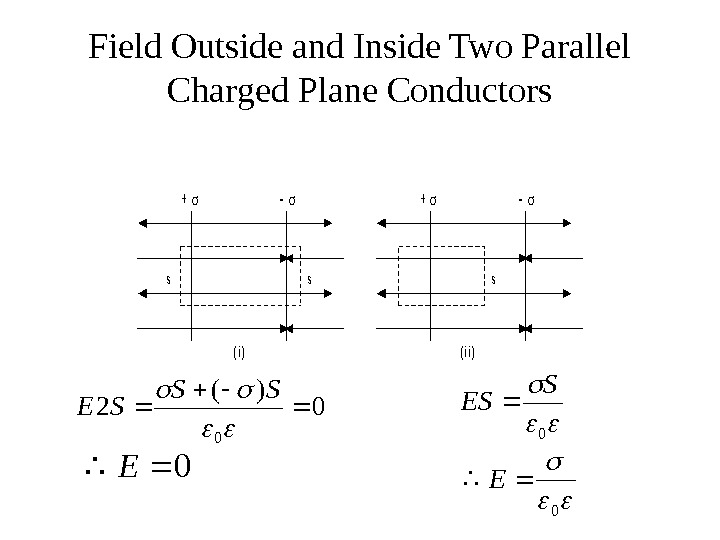Field Outside and Inside Two Parallel Charged Plane Conductors( i )( i i ) sss + + 0 )( 2 0 SS SE 0 E 0 S ES